Win up to 100% scholarship on Aakash BYJU'S JEE/NEET courses with ABNAT Win up to 100% scholarship on Aakash BYJU'S JEE/NEET courses with ABNAT

# JEE Main 2022 July 25 – Shift 2 Physics Question Paper with Solutions

The JEE Main 2022 July 25 – Shift 2 Physics Question Paper with Solutions is provided on this page. Students can solve and practise the JEE Main 2022 question paper with solutions and thus be familiar with the question pattern and difficulty level. The JEE Main 2022 answer keys contain the accurate solutions to the JEE Main questions. Students are recommended to revise and learn the JEE Main 2022 July 25 – Shift 2 Physics Question Paper with Solutions so that they can easily crack the problems for the upcoming JEE Main exam.

## JEE Main 2022 July 25th Shift 2 Physics Question Paper and Solutions

SECTION – A

Multiple Choice Questions: This section contains 20 multiple choice questions. Each question has 4 choices (1), (2), (3) and (4), out of which ONLY ONE is correct.

1. In AM modulation, a signal is modulated on a carrier wave such that maximum and minimum amplitudes are found to be 6 V and 2 V, respectively. The modulation index is

(A) 100%

(B) 80%

(C) 60%

(D) 50%

Sol. Amax = 6 V

Amin = 2 V

$$\begin{array}{l}\mu = \frac{A_{\text{max}}-A_{\text{min}}}{A_{\text{max}}+A_{\text{min}}}=\frac{6-2}{6+2}=0.5\end{array}$$

μ = 50 %

2. The electric current in a circular coil of 2 turns produces a magnetic induction B1 at its centre. The coil is unwound and is rewound into a circular coil of 5 turns and the same current produces a magnetic induction B2 at its centre. The ratio of B2/B1 is

$$\begin{array}{l}(\text{A})\ \frac{5}{2}\end{array}$$
$$\begin{array}{l}(\text{B})\ \frac{25}{4}\end{array}$$
$$\begin{array}{l}(\text{C})\ \frac{5}{4}\end{array}$$
$$\begin{array}{l}(\text{C})\ \frac{25}{2}\end{array}$$

Sol.

$$\begin{array}{l}B=\frac{n\mu_0I}{2R}\end{array}$$
$$\begin{array}{l}B_1=\frac{2\mu_0I}{2R_1}\end{array}$$
$$\begin{array}{l}B_2=\frac{5\mu_0I}{2R_2}\end{array}$$
$$\begin{array}{l}R_2=\frac{2R_1}{5}\end{array}$$
$$\begin{array}{l}\Rightarrow \frac{B_2}{B_1}=\frac{5}{2}\times \frac{R_1}{R_2}=\frac{25}{4}\end{array}$$

3. A drop of liquid of density ρ is floating half immersed in a liquid of density σ and surface tension 7.5 × 10–4 N cm–1. The radius of drop in cm will be (g = 10 ms–2)

$$\begin{array}{l}(\text{A})\frac{15}{\sqrt{(2\rho -\sigma)}}\end{array}$$
$$\begin{array}{l}(\text{B})\frac{15}{\sqrt{(\rho – \sigma)}}\end{array}$$
$$\begin{array}{l}(\text{C})\frac{3}{2\sqrt{(\rho – \sigma)}}\end{array}$$
$$\begin{array}{l}(\text{D})\frac{3}{20\sqrt{(2\rho – \sigma)}}\end{array}$$

Sol. Balancing the forces on drop

$$\begin{array}{l}2\pi RT=\frac{4}{3}\pi R^3\rho g = \frac{2}{3} \pi R^3 \sigma g \end{array}$$$$\begin{array}{l}\Rightarrow 2T =\frac{2R^2}{3}(\sigma – 2\rho)\times 10\end{array}$$
$$\begin{array}{l}\Rightarrow \frac{15 \times 10^{-2} \times 3}{10(\sigma – 2\rho)2}=R^2\end{array}$$
$$\begin{array}{l}R=\frac{3}{2 \times 10 }\sqrt{\frac{1}{(\sigma – 2 \rho)}}\end{array}$$
$$\begin{array}{l}=\frac{3}{20}\sqrt{\frac{1}{\sigma – 2\rho}}\ (\text{in m})\end{array}$$
$$\begin{array}{l}(R) \text{ in cm } = \frac{3 \times 100}{200}\sqrt{\frac{1}{\sigma – 2 \rho}}=15 \times \frac{1}{\sqrt{\sigma – 2 \rho}}\end{array}$$
$$\begin{array}{l}\text{Now if }2\rho > \sigma(R_{\text{in cm}}) = \frac{15}{\sqrt{2\rho – \sigma}}\end{array}$$

4. Two billiard balls of mass 0.05 kg each moving in opposite directions with 10 ms–1 collide and rebound with the same speed. If the time duration of contact is t = 0.005 s, then what is the force exerted on the ball due to each other?

(A) 100 N

(B) 200 N

(C) 300 N

(D) 400 N

Sol. Change in momentum of one ball

= 2 × (0.05)(10) kg m/s

= 1 kg m/s

$$\begin{array}{l}\Rightarrow F_{\text{avg}} = \frac{1}{\Delta t}=\frac{1}{0.005} \text{ N}\end{array}$$

= 200 N

5. For a free body diagram shown in the figure, the four forces are applied in the ‘x’ and ‘y’ directions. What additional force must be applied and at what angle with positive x-axis so that net acceleration of body is zero?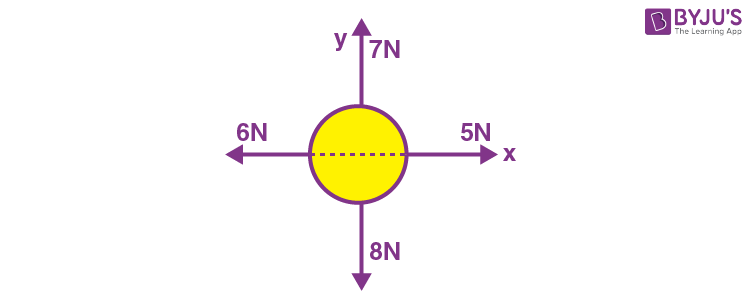$$\begin{array}{l}(\text{A})\ \sqrt{2} \text{ N}, 45^{\circ}\end{array}$$
$$\begin{array}{l}(\text{B})\ \sqrt{2} \text{ N}, 135^{\circ}\end{array}$$
$$\begin{array}{l}(\text{C})\ \frac{2}{\sqrt{3}}\text{ N}, 30^{\circ}\end{array}$$

(D) 2 N, 45°

Sol. Resultant of already applied forces

$$\begin{array}{l}=-\hat{i}-\hat{j}\end{array}$$

⇒ Force required to balance

$$\begin{array}{l}=\hat{i}+\hat{j}\end{array}$$

⇒ Force required

$$\begin{array}{l}=\sqrt{2} \text{ N}\end{array}$$
in magnitude at angle 45° with +ve x-axis

6. Capacitance of an isolated conducting sphere of radius R1 becomes n times when it is enclosed by a concentric conducting sphere of radius R2 connected to earth. The ratio of their radii (R2/R1) is:

$$\begin{array}{l}(\text{A})\ \frac{n}{n-1}\end{array}$$
$$\begin{array}{l}(\text{B})\ \frac{2n}{2n+1}\end{array}$$
$$\begin{array}{l}(\text{C})\ \frac{n+1}{n}\end{array}$$
$$\begin{array}{l}(\text{D})\ \frac{2n+1}{n}\end{array}$$

Sol. Initially = C0 = 4πε0R1

finally

$$\begin{array}{l}\frac{4\pi \varepsilon_0R_1R_2 }{R_2-R_1} = nC_0 = 4\pi\varepsilon_0nR_1\end{array}$$
$$\begin{array}{l}\frac{R_2}{R_2-R_1}=n\end{array}$$
$$\begin{array}{l}1-\frac{R_1}{R_2}=\frac{1}{n}\end{array}$$
$$\begin{array}{l}\frac{R_1}{R_2}=\frac{n-1}{n}\end{array}$$
$$\begin{array}{l}\frac{R_2}{R_1}=\frac{n}{n-1}\end{array}$$

7. The ratio of wavelengths of proton and deuteron accelerated by potential Vp and Vd is 1 : √2. Then, the ratio of Vp to Vd will be:

(A) 1 : 1

$$\begin{array}{l}(\text{B})\ \sqrt{2}:1\end{array}$$

(C) 2 : 1

(D) 4 : 1

Sol.

$$\begin{array}{l}\lambda = \frac{h}{mv}=\frac{h}{\sqrt{2meV}}\end{array}$$
$$\begin{array}{l}\text{so }\frac{\lambda_p}{\lambda_d}=\frac{\sqrt{m_dV_d}}{\sqrt{m_pV_p}}=\frac{1}{\sqrt{2}}\end{array}$$
$$\begin{array}{l}\frac{2V_d}{V_p}=\frac{1}{2}\end{array}$$
$$\begin{array}{l}\frac{V_p}{V_d}=\frac{4}{1}\end{array}$$

8. For an object placed at a distance 2.4 m from a lens, a sharp focused image is observed on a screen placed at a distance 12 cm form the lens. A glass plate of refractive index 1.5 and thickness 1 cm is introduced between lens and screen such that the glass plate plane faces parallel to the screen. By what distance should the object be shifted so that a sharp focused image is observed again on the screen?

(A) 0.8 m

(B) 3.2 m

(C) 1.2 m

(D) 5.6 m

Sol. The shift produced by the glass plate is

$$\begin{array}{l}d=t\left(1-\frac{1}{\mu}\right)=1\times \left(1-\frac{1}{1.5}\right)=\frac{1}{3} \text{ cm}\end{array}$$

So final image must be produced at

$$\begin{array}{l}\left(12-\frac{1}{3}\right)\text{ cm} = \frac{35}{3}\text{ cm}\end{array}$$
from lens so that glass plate must shift it to produce image at screen. So

$$\begin{array}{l}\frac{1}{12}-\frac{1}{-240}=\frac{1}{f}=\frac{1}{35/3}-\frac{1}{u}\end{array}$$
$$\begin{array}{l}\frac{1}{u}=\frac{3}{35}-\frac{1}{12}-\frac{1}{240}\end{array}$$

or u = – 560 cm

so shift = 5.6 – 2.4 = 3.2 m

9. Light wave traveling in air along x-direction is given by Ey = 540 sin π × 104 (xct)Vm–1. Then, the peak value of magnetic field of wave will be (Given c = 3 × 108 ms–1)

(A) 18 × 10–7 T

(B) 54 × 10–7 T

(C) 54 × 10–8 T

(D) 18 × 10–8 T

Sol. c = 3 × 108 m/sec

$$\begin{array}{l}B=\frac{E}{c}=\frac{540}{3 \times 10^8}=18 \times 10^{-7} \text{ T}\end{array}$$

10. When you walk through a metal detector carrying a metal object in your pocket, it raises an alarm. This phenomenon works on:

(A) Electromagnetic induction

(B) Resonance in ac circuits

(C) Mutual induction in ac circuits

(D) Interference of electromagnetic waves

Sol. Metal detector works on the principle of resonance in ac circuits.

11. An electron with energy 0.1 keV moves at right angle to the earth’s magnetic field of 1 × 10–4 Wbm–2. The frequency of revolution of the electron will be

(Take mass of electron = 9.0 × 10–31 kg)

(A) 1.6 × 105 Hz

(B) 5.6 × 105 Hz

(C) 2.8 × 106 Hz

(D) 1.8 × 106 Hz

Sol.

$$\begin{array}{l}T=\frac{2\pi m}{Bq}\end{array}$$
$$\begin{array}{l}\Rightarrow \text{ Frequency }f = \frac{Bq}{2\pi m}\end{array}$$
$$\begin{array}{l}=\frac{10^{-4}\times 1.6 \times 10^{-19}}{2\pi \times 9 \times 10^{-31}}\end{array}$$
$$\begin{array}{l} \simeq2.8\times10^6 ~\text{Hz}\end{array}$$

12. A current of 15 mA flows in the circuit as shown in figure. The value of potential difference between the points A and B will be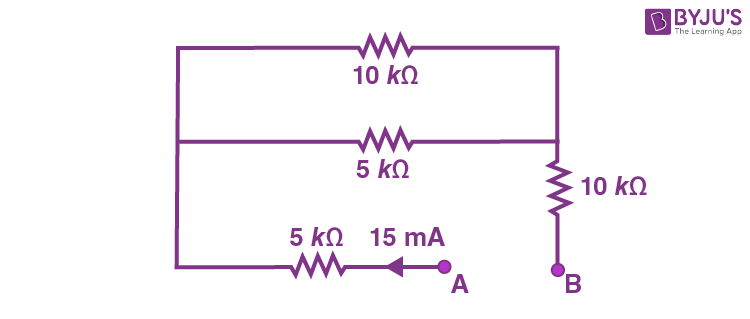(A) 50 V

(B) 75 V

(C) 150 V

(D) 275 V

Sol. Effective

$$\begin{array}{l}R=\left[5 + \frac{5 \times 10}{5+10} + 10 \right] \text{ k}\Omega\end{array}$$
$$\begin{array}{l}=\frac{275}{15} \text{ k}\Omega\end{array}$$
$$\begin{array}{l}\Rightarrow \ \Delta V_{AB} = 15 \text{ mA}\times \frac{275}{15}\text{ k}\Omega = 275 ~\text{V}\end{array}$$

13. The length of a seconds pendulum at a height h = 2R from earth surface will be

(Given R = Radius of earth and acceleration due to gravity at the surface of earth, g = π2 ms–2)

$$\begin{array}{l}(\text{A})\ \frac{2}{9} \text{ m}\end{array}$$
$$\begin{array}{l}(\text{B})\ \frac{4}{9} \text{ m}\end{array}$$
$$\begin{array}{l}(\text{C})\ \frac{8}{9} \text{ m}\end{array}$$
$$\begin{array}{l}(\text{D})\ \frac{1}{9} \text{ m}\end{array}$$

Sol.

$$\begin{array}{l}g=\frac{GM}{(R+h)^2}=\frac{GM}{9R^2}=\frac{g_0}{9}\end{array}$$

$$\begin{array}{l}\Rightarrow T=2\pi \sqrt \frac{l}{g}=2\pi \sqrt \frac{l}{\frac{g_0}{9}} \end{array}$$
$$\begin{array}{l}\Rightarrow 2=2\pi \sqrt \frac{9l}{g_0}\end{array}$$
$$\begin{array}{l}\Rightarrow l=\frac{g_0}{9\pi^2}= \frac{1}{9}m\end{array}$$

14. Sound travels in a mixture of two moles of helium and n moles of hydrogen. If rms speed of gas molecules in the mixture is √2 times the speed of sound, then the value of n will be

(A) 1

(B) 2

(C) 3

(D) 4

Sol. Molar mass

$$\begin{array}{l}M=\frac{2 \times 4 + n \times 1}{2 + n}~~~~~~…(i)\end{array}$$
$$\begin{array}{l}\text{Also, }\gamma = \frac{n_1C_{P_1}+n_2C_{P_2}}{n_1C_{V_1} + n_2C_{V_2}} = \frac{2\times 5R + n\times 7R}{2\times 3R + n \times 5R}\end{array}$$
$$\begin{array}{l}\Rightarrow\gamma = \frac{10 + 7n}{6+5n}~~~~~…(ii)\end{array}$$

Given that

$$\begin{array}{l}V_{\text{rms}} = \sqrt{2}V_{\text{sound}}\end{array}$$
$$\begin{array}{l}\Rightarrow \sqrt{\frac{3RT}{M}}=\sqrt{2}\sqrt{\frac{\gamma RT}{M}}\end{array}$$
$$\begin{array}{l}\Rightarrow \gamma = \frac{3}{2}\end{array}$$

n = 2

15. Let η1 is the efficiency of an engine at T1 = 447°C and T2 = 147°C while η2 is the efficiency at T1 = 947°C and T2 = 47°C.

$$\begin{array}{l}\text{The ratio}\ \frac{\eta_1 }{\eta_2}\ \text{will be}\end{array}$$

(A) 0.41

(B) 0.56

(C) 0.73

(D) 0.70

Sol.

$$\begin{array}{l}\eta_1 = 1-\frac{420}{720}=\frac{300}{720}\end{array}$$

And

$$\begin{array}{l}\eta_2 = 1-\frac{320}{1220}=\frac{900}{1220}\end{array}$$
$$\begin{array}{l}\Rightarrow \frac{\eta_1}{\eta_2} = \frac{300}{720}\times \frac{1220}{900}\simeq0.56\end{array}$$

16. An object is taken to a height above the surface of earth at a distance (5/4)R from the centre of the earth. Where radius of earth, R = 6400 km. The percentage decrease in the weight of the object will be

(A) 36%

(B) 50%

(C) 64%

(D) 25%

Sol. w = mg

$$\begin{array}{l}w’ =\frac{mg}{\left( 1+\frac{h}{R}\right)^2}=\frac{mg}{\left(\frac{5}{4}\right)^2}=\frac{16}{25}mg\end{array}$$

∴ Percent decrease in weight

$$\begin{array}{l}=\left(1-\frac{16}{25}\right)\times 100\% = 36\%\end{array}$$

17. A bag of sand of mass 9.8 kg is suspended by a rope. A bullet of 200 g travelling with speed 10 ms–1 gets embedded in it, then loss of kinetic energy will be

(A) 4.9 J

(B) 9.8 J

(C) 14.7 J

(D) 19.6 J

Sol. Loss in KE

$$\begin{array}{l} = \frac{1}{2}\times \frac{m_1m_2}{m_1 + m_2} \times v^2\end{array}$$
$$\begin{array}{l}=\frac{1}{2}\times \frac{9.8 \times 0.2}{10}\times(10)^2 =9.8 ~\text{J}\end{array}$$

18. A ball is projected from the ground with a speed 15 ms–1 at an angle θ with horizontal so that its range and maximum height are equal, then ‘tan θ’ will be equal to

$$\begin{array}{l}(\text{A})\ \frac{1}{4}\end{array}$$
$$\begin{array}{l}(\text{B})\ \frac{1}{2}\end{array}$$

(C) 2

(D) 4

Sol.

$$\begin{array}{l}\because \tan \theta = \frac{4H}{R}\end{array}$$

⇒ tanθ = 4 × 1

⇒ tanθ = 4

19. The maximum error in the measurement of resistance, current and time for which current flows in an electrical circuit are 1%, 2% and 3% respectively. The maximum percentage error in the detection of the dissipated heat will be

(A) 2

(B) 4

(C) 6

(D) 8

Sol. H = i2Rt

∵ % error in H = 2 × 2% + 1% + 3%

= 8%

20. Hydrogen atom from excited state comes to the ground state by emitting a photon of wavelength λ. The value of principal quantum number ‘n’ of the excited state will be, (R: Rydberg constant)

$$\begin{array}{l}(\text{A})\ \sqrt{\frac{\lambda R}{\lambda – 1}}\end{array}$$
$$\begin{array}{l}(\text{B})\ \sqrt{\frac{\lambda R}{\lambda R – 1}}\end{array}$$
$$\begin{array}{l}(\text{C})\ \sqrt{\frac{\lambda}{\lambda R – 1}}\end{array}$$
$$\begin{array}{l}(\text{D})\ \sqrt{\frac{\lambda R^2}{\lambda R – 1}}\end{array}$$

Sol.

$$\begin{array}{l}\because \frac{1}{\lambda} = R\left(\frac{1}{1^2}-\frac{1}{n^2}\right)\end{array}$$
$$\begin{array}{l}\Rightarrow \frac{1}{\lambda R}=1-\frac{1}{n^2}\end{array}$$
$$\begin{array}{l}\Rightarrow \frac{1}{n^2}=1-\frac{1}{\lambda R}=\frac{\lambda R – 1}{\lambda R}\end{array}$$
$$\begin{array}{l}\Rightarrow n = \sqrt{\frac{\lambda R}{\lambda R-1}}\end{array}$$

SECTION – B

Numerical Value Type Questions: This section contains 10 questions. In Section B, attempt any five questions out of 10. The answer to each question is a NUMERICAL VALUE. For each question, enter the correct numerical value (in decimal notation, truncated/rounded-off to the second decimal place; e.g. 06.25, 07.00, –00.33, –00.30, 30.27, –27.30) using the mouse and the on-screen virtual numeric keypad in the place designated to enter the answer.

1. A particle is moving in a straight line such that its velocity is increasing at 5 ms–1 per meter. The acceleration of the particle is _______ms–2 at a point where its velocity is 20 ms–1.

Sol.

$$\begin{array}{l}\frac{dv}{dx}=5 \text{ ms}^{-1}/\text{m}\end{array}$$

Acceleration of particle

when v = 20 m/s

$$\begin{array}{l}a=v\frac{dv}{dx}=20(5) \text{ m/s}^{2} = 100 \text{ m/s}^{2}\end{array}$$

2. Three identical spheres each of mass M are placed at the corners of a right angled triangle with mutually perpendicular sides equal to 3 m each. Taking point of intersection of mutually perpendicular sides as origin, the magnitude of position vector of centre of mass of the system will be √x m. The value of x is _______ .

Sol.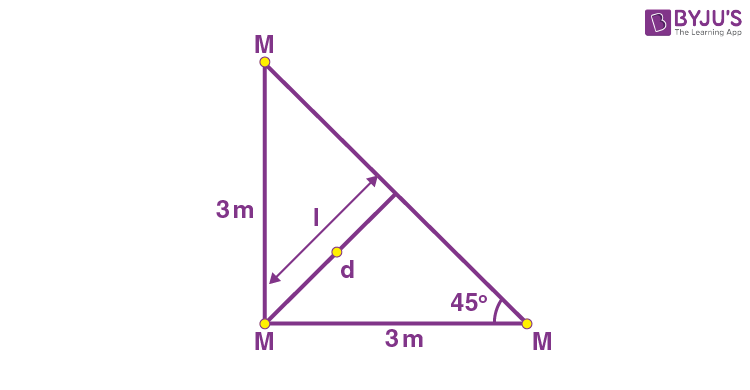$$\begin{array}{l}d_{\text{cm}} = 3 \sin 45^{\circ} = \frac{3}{\sqrt{2}} \end{array}$$
$$\begin{array}{l}d_{\text{cm}} = \frac{2}{3}\times \frac{3}{\sqrt{2}} = \sqrt{2} = \sqrt{x} \\x = 2\end{array}$$

3. A block of ice of mass 120 g at temperature 0°C is put in 300 g of water at 25°C. The x g of ice melts as the temperature of the water reaches 0°C. The value of x is _______.

[Use specific heat capacity of water = 4200 Jkg–1K–1, Latent heat of ice = 3.5 ×105 Jkg–1]

Heat lost by water = Heat gained by ice

0.3 × 4200 × 25 = x × 3.5 × 105

$$\begin{array}{l}x=\frac{0.3\times 4200 \times 25}{3.5 \times 10^5}\end{array}$$

= 90 × 100 × 105 × 103 gram = 90 gm

4. x/(x + 4) is the ratio of energies of photons produced due to transition of an electron of hydrogen atom from its

(i) Third permitted energy level to the second level and

(ii) The highest permitted energy level to the second permitted level.

The value of x will be ______.

Sol.

$$\begin{array}{l}E_n = -\frac{13.6}{n^2} \text{ eV}\end{array}$$
$$\begin{array}{l}\frac{\frac{1}{2^2}-\frac{1}{3^2}}{\frac{1}{2^2}}=\frac{x}{x+4}\end{array}$$
$$\begin{array}{l}\Rightarrow \frac{9-4}{9\times 4\times \frac{1}{4}}=\frac{x}{x+4}=\frac{5}{9}\\ x = 5\end{array}$$

5. In a potentiometer arrangement, a cell of emf 1.20 V gives a balance point at 36 cm length of wire. This cell is now replaced by another cell of emf 1.80 V. The difference in balancing length of potentiometer wire in above conditions will be _______ cm.

Sol. E l

$$\begin{array}{l}\frac{1.2}{1.8}=\frac{36}{l’}\end{array}$$
$$\begin{array}{l}l’= \frac{3}{2}\times 36 = 54 \text{ cm}\end{array}$$

Δl = l‘ – l = 54 – 36 = 18 cm

6. Two ideal diodes are connected in the network as shown is figure. The equivalent resistance between A and B is ___ Ω.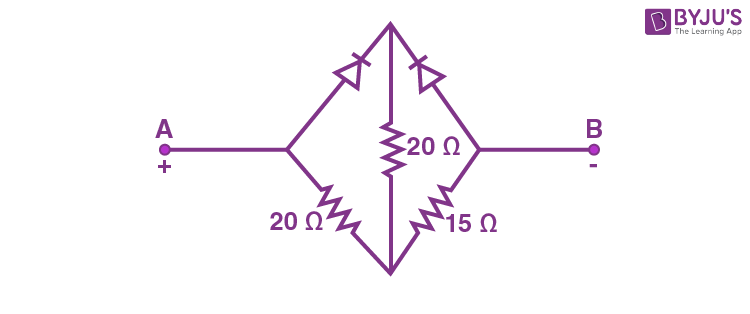Sol.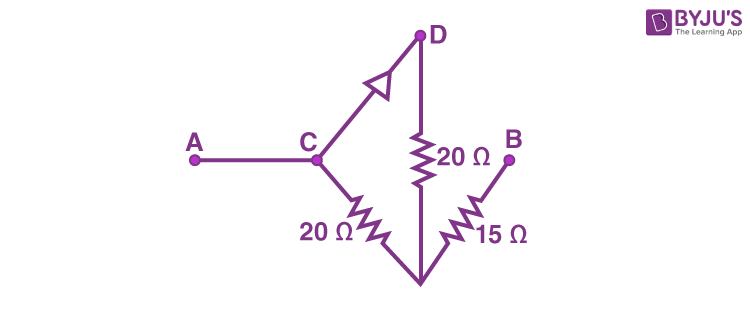$$\begin{array}{l}R = \frac{20 \times 20}{40}+15 = 25\ \Omega\end{array}$$

7. Two waves executing simple harmonic motions travelling in the same direction with same amplitude and frequency are superimposed. The resultant amplitude is equal to the √3 times of amplitude of individual motions. The phase difference between the two motions is____ (degree).

Sol.

$$\begin{array}{l}A_{\text{net}} = \sqrt{A_1^2+A_2^2+2A_1A_2 \cos \phi}\end{array}$$
$$\begin{array}{l}\sqrt{3}A = \sqrt{A^2+A^2 + 2A^2\cos \phi}\end{array}$$
$$\begin{array}{l}3A^2 = 2A^2 + 2A^2 \cos \phi\end{array}$$
$$\begin{array}{l}\cos \phi = \frac{1}{2}\end{array}$$
$$\begin{array}{l}\phi = 60^{\circ}\end{array}$$

8. Two parallel plate capacitors of capacity C and 3C are connected in parallel combination and charged to a potential difference 18 V. The battery is then disconnected and the space between the plates of the capacitor of capacity C is completely filled with a material of dielectric constant 9. The final potential difference across the combination of capacitors will be ____ V.

Sol.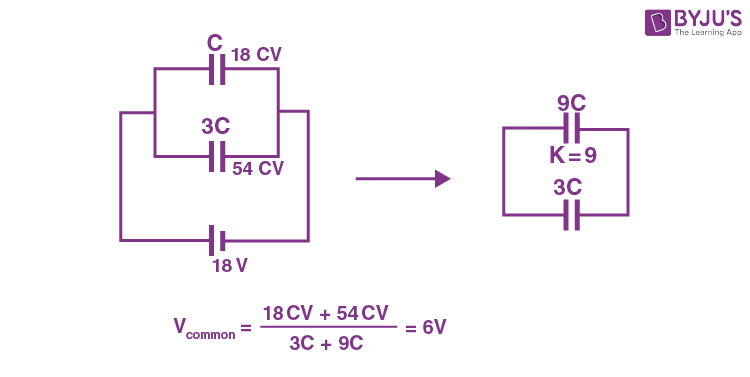9. A convex lens of focal length 20 cm is placed in front of a convex mirror with principal axis coinciding each other. The distance between the lens and mirror is 10 cm. A point object is placed on principal axis at a distance of 60 cm from the convex lens. The image formed by combination coincides the object itself. The focal length of the convex mirror is_______cm.

Sol.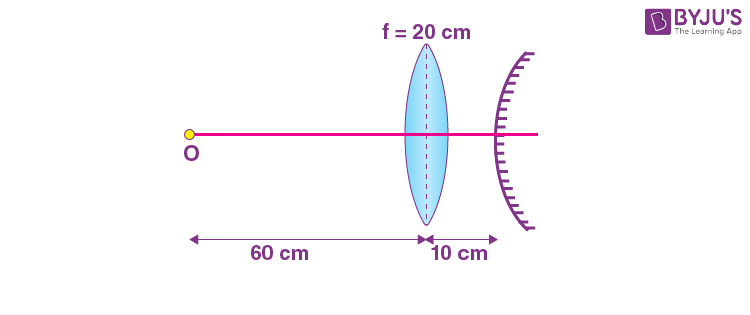$$\begin{array}{l}\frac{1}{v}+\frac{1}{u}=\frac{1}{f}\end{array}$$
$$\begin{array}{l} \frac{1}{v}-\frac{1}{-60}=\frac{1}{20}\end{array}$$
$$\begin{array}{l} \frac{1}{v}=-\frac{1}{60}+\frac{1}{20}=\frac{-1+3}{60}=\frac{2}{60}\end{array}$$

v = +30 cm

∴ Radius of curvature of mirror = 30 – 10 = 20 cm

$$\begin{array}{l} \Rightarrow f_{\text{mirror}} = \frac{20}{2}= 10 \text{ cm}\end{array}$$

10. Magnetic flux (in weber) in a closed circuit of resistance 20 Ω varies with time t(s) as φ = 8t2 – 9t + 5. The magnitude of the induced current at t = 0.25 s will be _____ mA.

Sol. R = 20 Ω

φ = 8t2 – 9t + 5

$$\begin{array}{l} \varepsilon = \left|-\frac{d \phi}{dt}\right| = |16t-9|=|16(0.25)-9|=5\end{array}$$
$$\begin{array}{l} i=\frac{\varepsilon}{R}=\frac{5}{20}=0.25 \text{ A} = \frac{0.25}{10^3}\times 10^3 \text{ A}=250 \text{ mA}\end{array}$$

### Download PDF of JEE Main 2022 July 25 Shift 2 Physics Paper & Solutions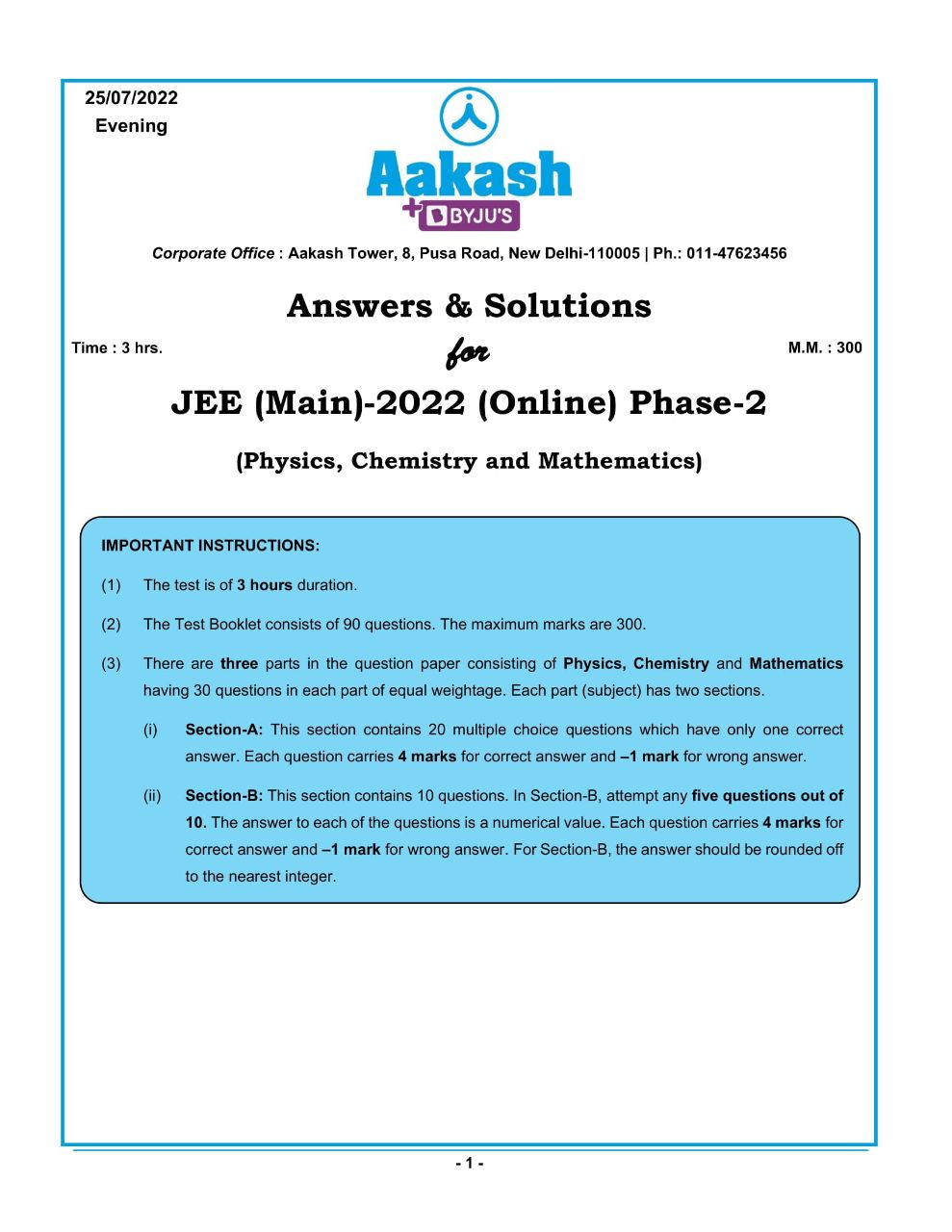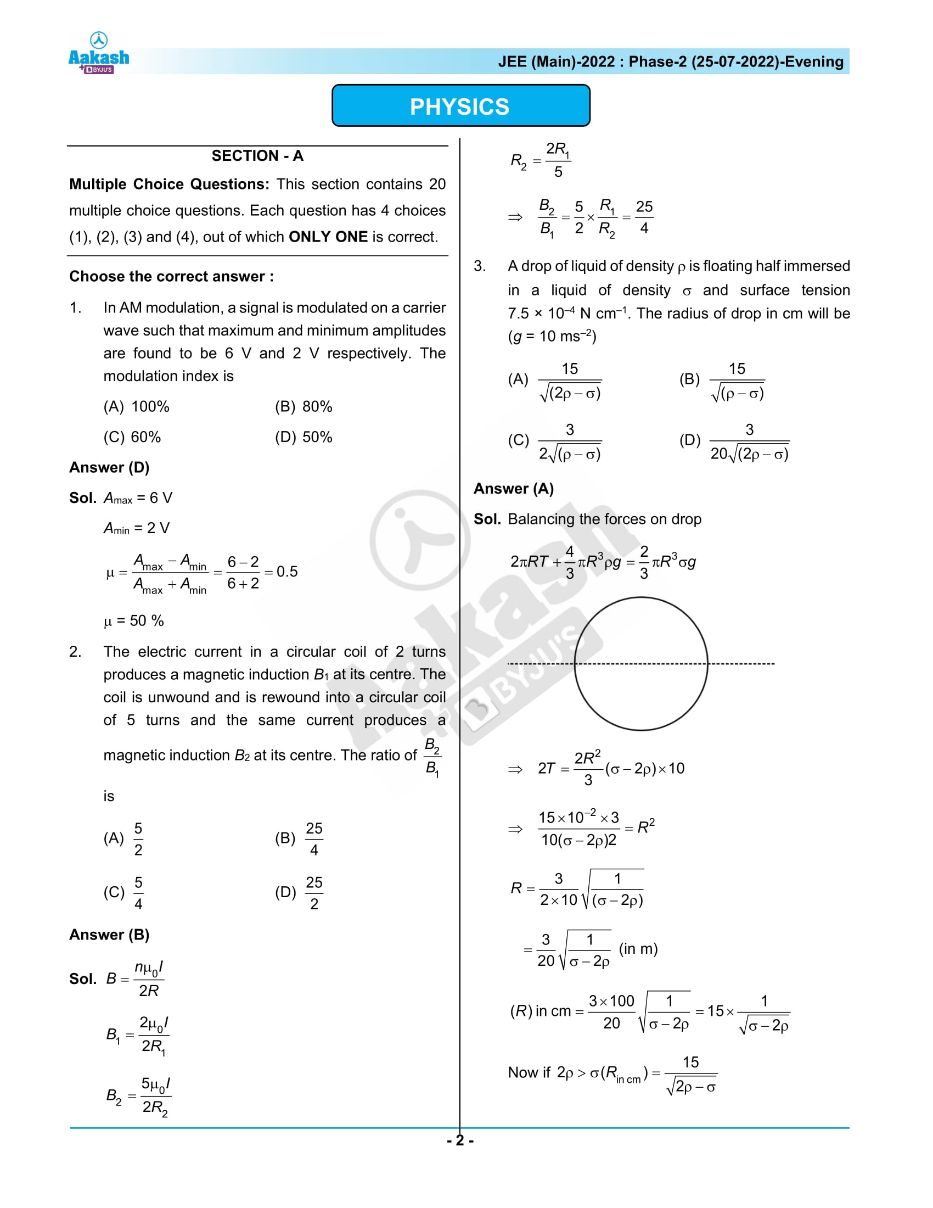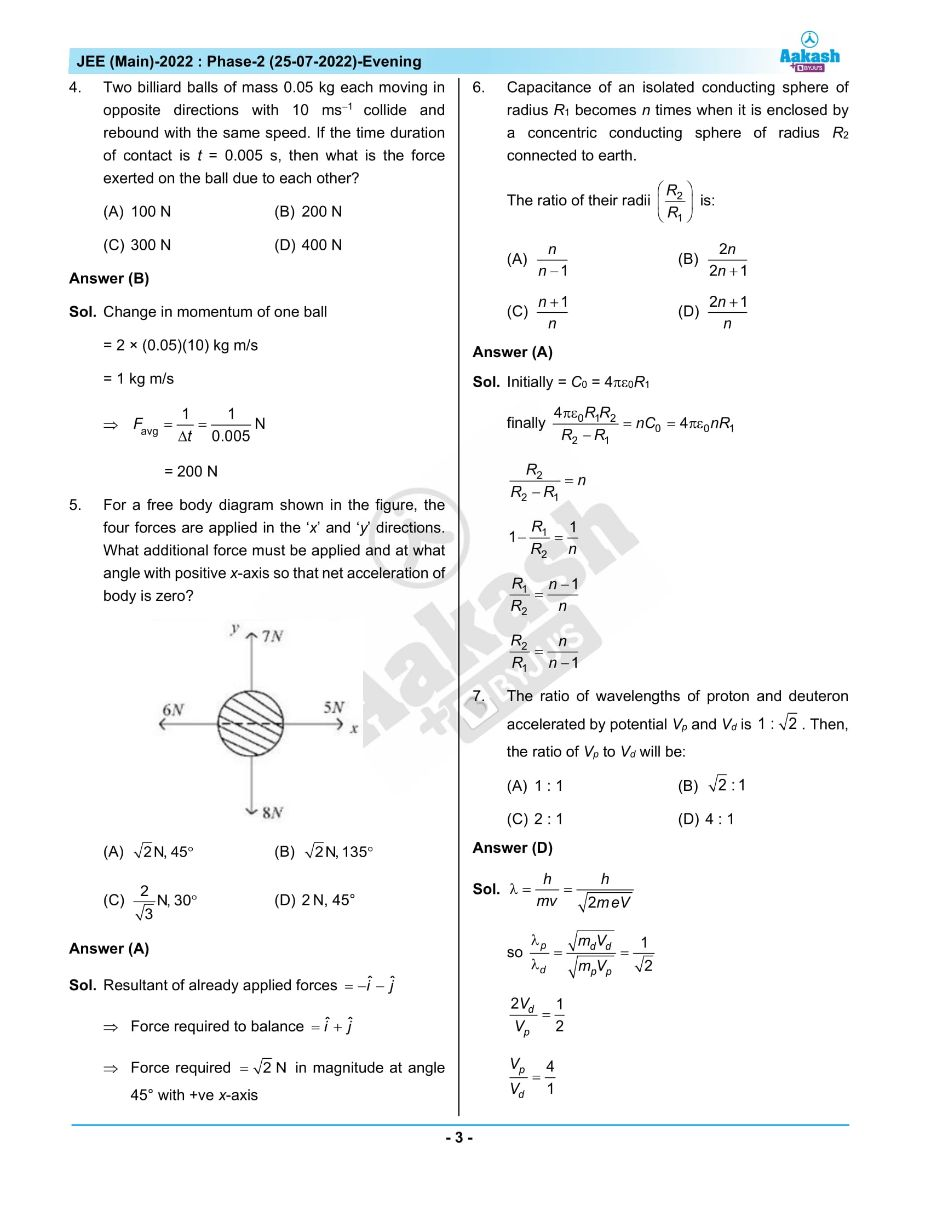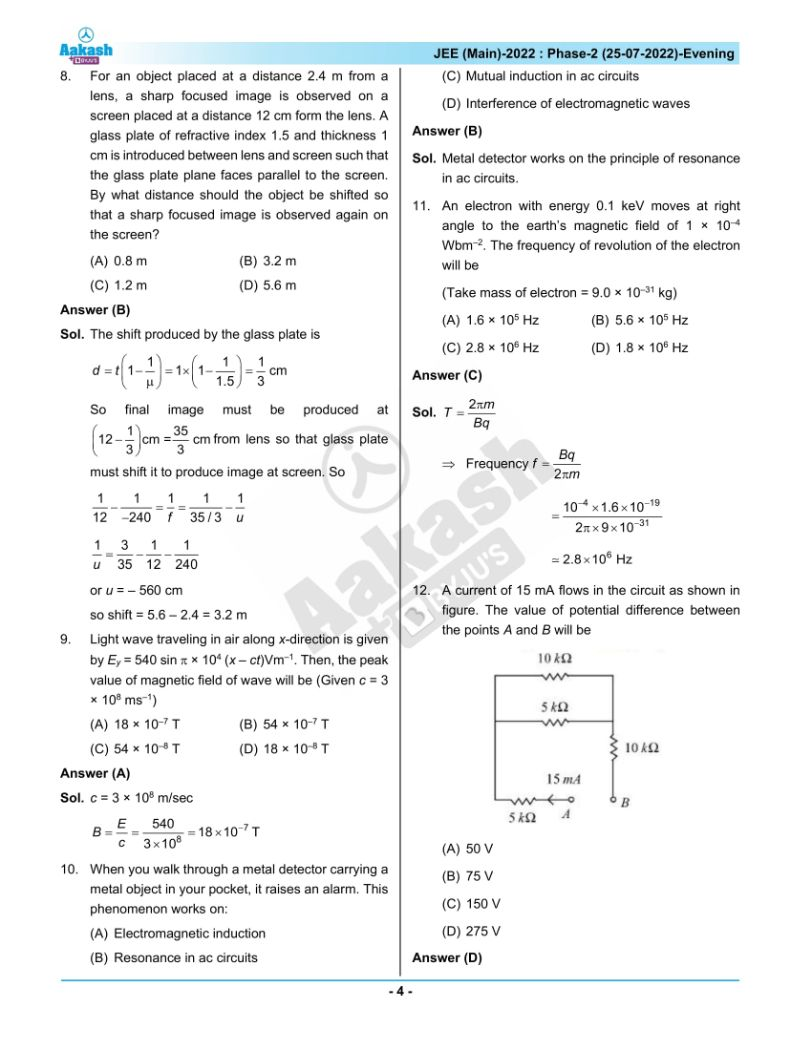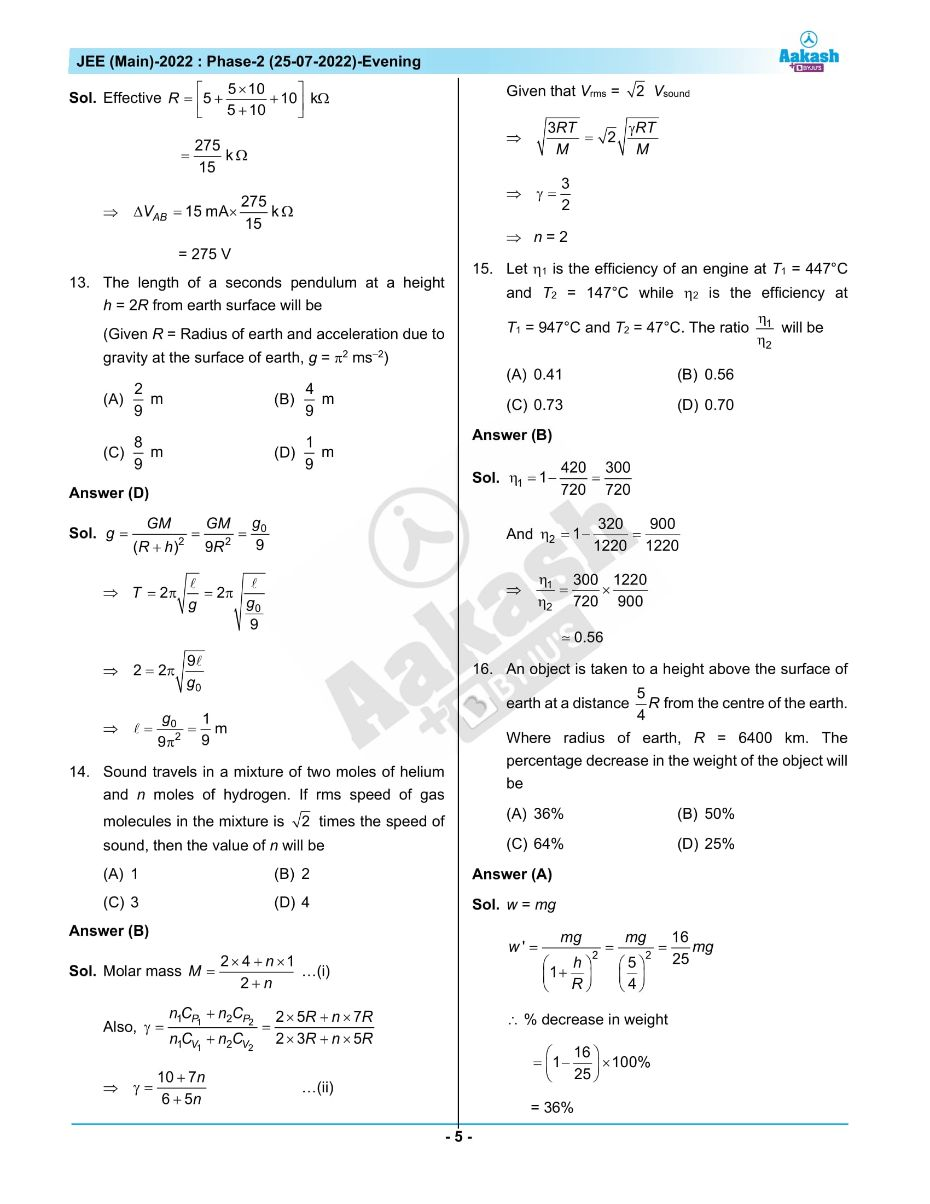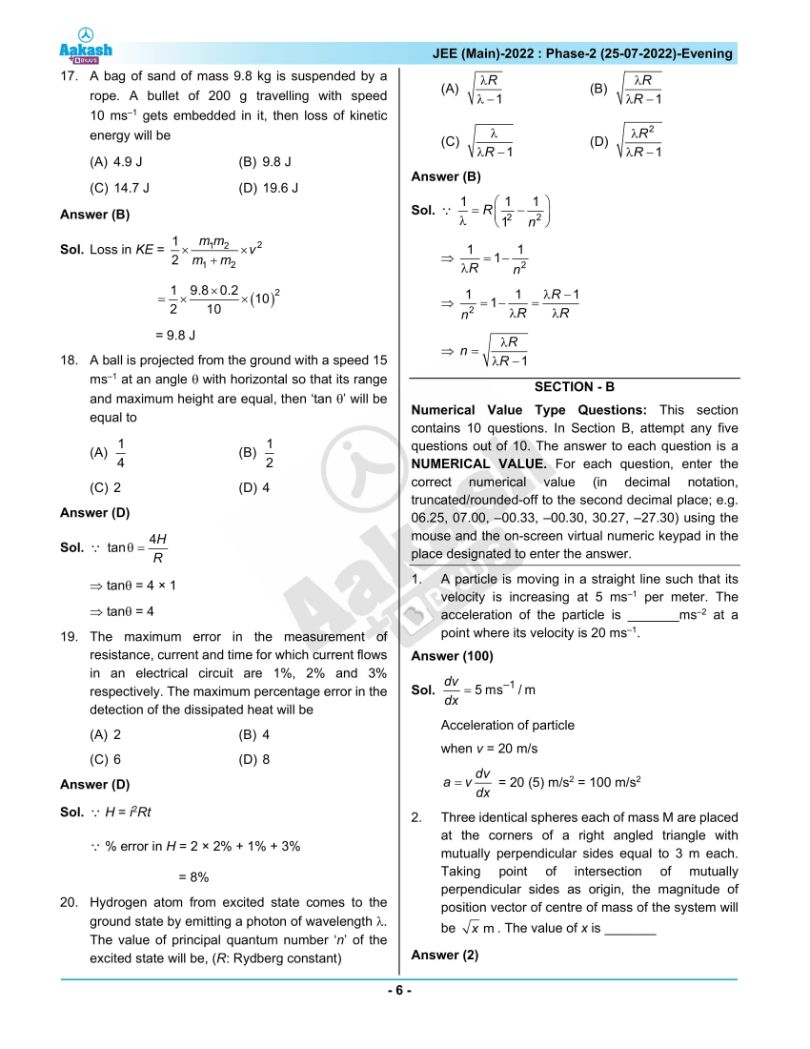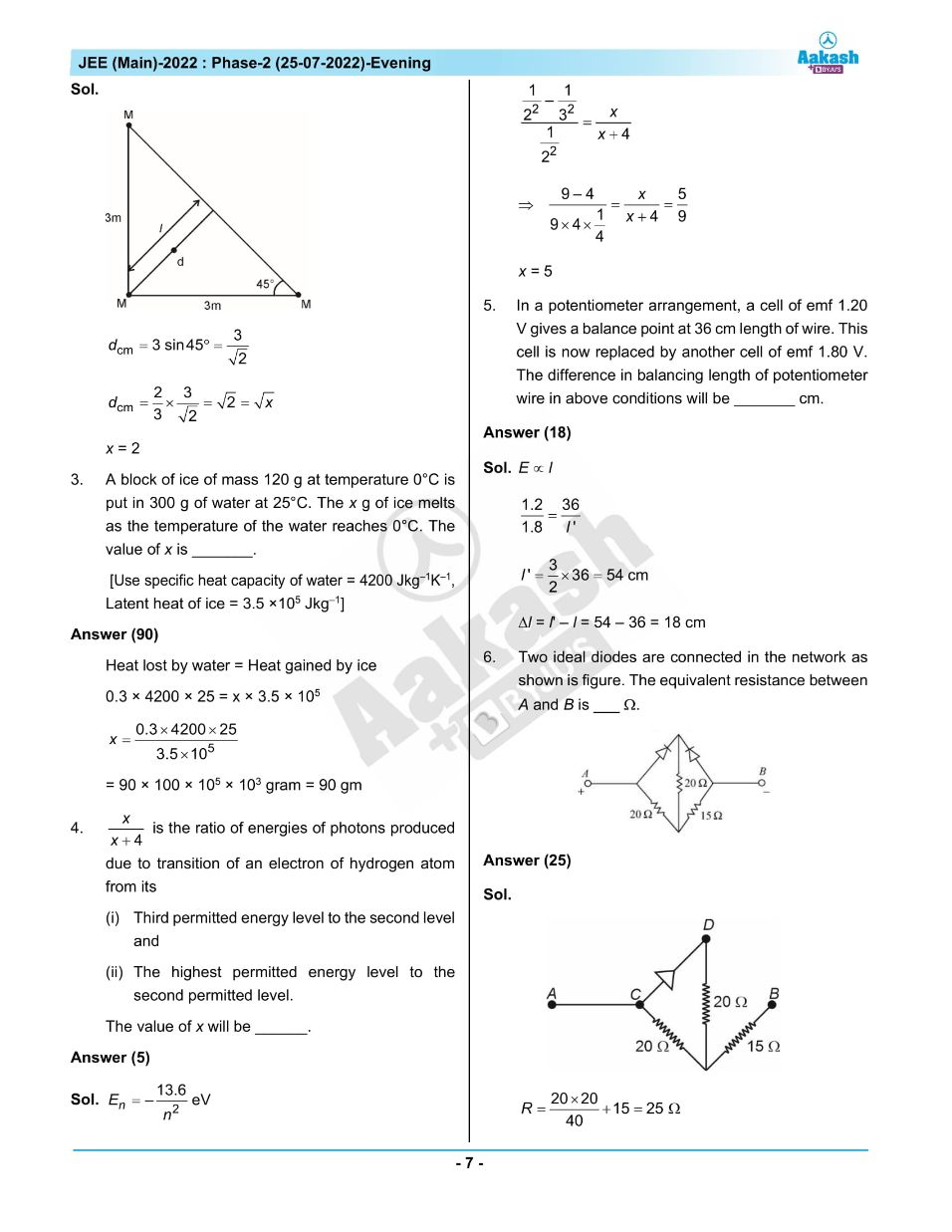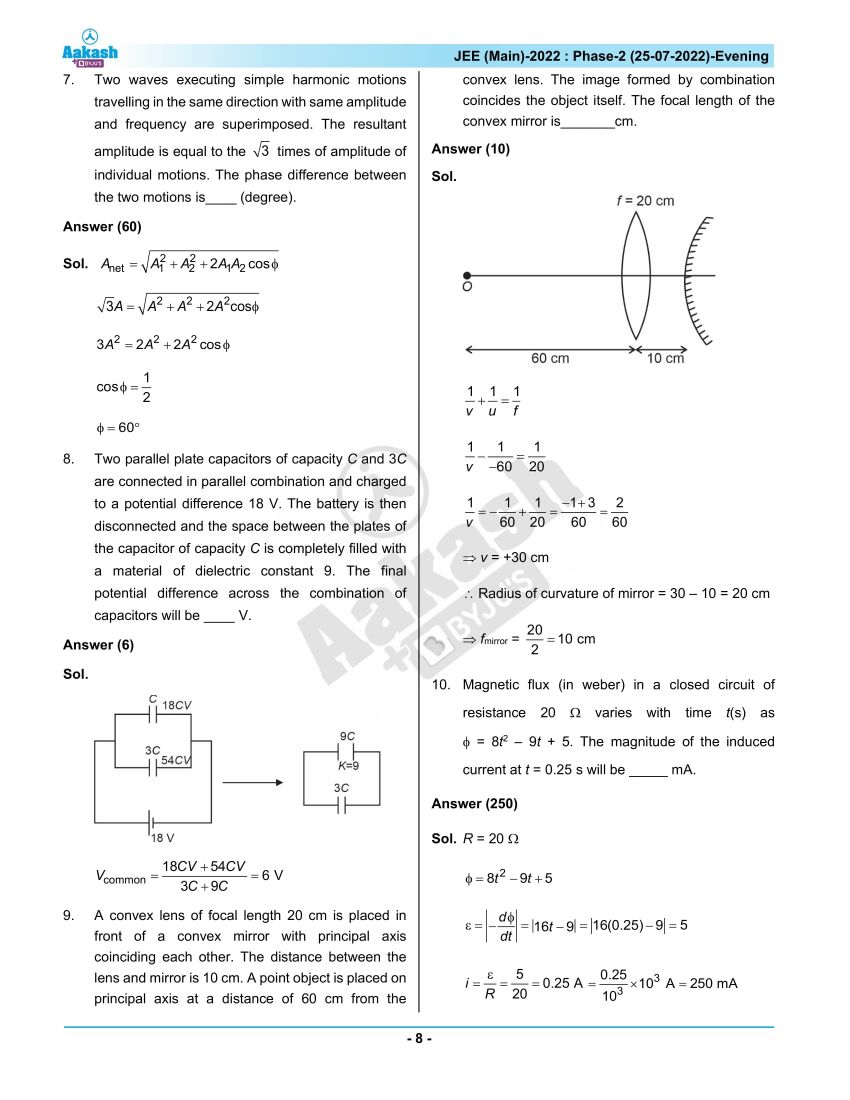## JEE Main 2022 July 25th Shift 2 Paper Analysis